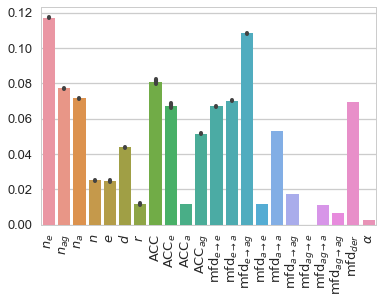# Application 1: ProvStore Documents¶

Identifying owners of provenance documents from their provenance network metrics

• Goal: To determine if the provenance network analytics method can identify the owner of a provenance document from its provenance network metrics.
• Training data: In order to ensure that there are sufficient samples to represent a user's provenance documents the Training phase, we limit our experiment to users who have at least 20 documents. There are fourteen such users (the authors were excluded to avoid bias), who we named $u_{1}, u_{2}, \ldots, u_{14}$. Their numbers of documents range between 21 and 6,745, with the total number of documents in the data set is 13,870.
• Classification labels: $\mathcal{L} = \left\{ u_1, u_2, \ldots, u_{14} \right\}$, where $l_{x} = u_i$ if the provenance document $x$ belongs to user $u_i$. Hence, there are 14 labels in total.

For each provenance document, we calculate the 22 provenance network metrics. The dataset provided contains those metrics values for 13,870 provenance documents along with the owner identifier (i.e. $u_{1}, u_{2}, \ldots, u_{14}$).

In :
import pandas as pd

In :
df = pd.read_csv("provstore/data.csv")

Out:
label entities agents activities nodes edges diameter assortativity acc acc_e ... mfd_e_a mfd_e_ag mfd_a_e mfd_a_a mfd_a_ag mfd_ag_e mfd_ag_a mfd_ag_ag mfd_der powerlaw_alpha
0 u_3 17 5 9 31 49 6 -0.196362 0.444709 0.466667 ... 5 8 4 2 5 0 0 0 3 -1.0
1 u_2 7 0 2 9 0 -1 -1.000000 0.000000 0.000000 ... 0 0 0 0 0 0 0 0 -1 -1.0
2 u_2 7 0 2 9 0 -1 -1.000000 0.000000 0.000000 ... 0 0 0 0 0 0 0 0 -1 -1.0
3 u_2 7 0 2 9 0 -1 -1.000000 0.000000 0.000000 ... 0 0 0 0 0 0 0 0 -1 -1.0
4 u_2 7 0 2 9 0 -1 -1.000000 0.000000 0.000000 ... 0 0 0 0 0 0 0 0 -1 -1.0

5 rows × 23 columns

In :
df.describe()

Out:
entities agents activities nodes edges diameter assortativity acc acc_e acc_a ... mfd_e_a mfd_e_ag mfd_a_e mfd_a_a mfd_a_ag mfd_ag_e mfd_ag_a mfd_ag_ag mfd_der powerlaw_alpha
count 13870.000000 13870.00000 13870.000000 13870.000000 13870.000000 13870.000000 13870.000000 13870.000000 13870.000000 13870.000000 ... 13870.000000 13870.000000 13870.000000 13870.000000 13870.000000 13870.000000 13870.000000 13870.000000 13870.000000 13870.000000
mean 9.913338 2.08695 1.836193 13.836482 19.212689 0.868926 -0.628690 0.347835 0.341142 0.323606 ... 1.312761 1.754939 1.073540 0.709229 0.752127 0.017448 0.014924 0.030353 2.185436 -0.916534
std 28.931915 2.27716 18.570823 43.352894 134.640366 1.943905 0.376718 0.394531 0.409577 0.395727 ... 1.769329 1.314874 1.622606 1.343363 1.077628 0.200902 0.152351 0.209759 5.211118 0.612437
min 0.000000 0.00000 0.000000 1.000000 0.000000 -1.000000 -1.000000 0.000000 0.000000 0.000000 ... 0.000000 0.000000 0.000000 0.000000 0.000000 0.000000 0.000000 0.000000 -1.000000 -1.000000
25% 2.000000 1.00000 0.000000 5.000000 5.000000 -1.000000 -1.000000 0.000000 0.000000 0.000000 ... 0.000000 1.000000 0.000000 0.000000 0.000000 0.000000 0.000000 0.000000 1.000000 -1.000000
50% 4.000000 1.00000 1.000000 7.000000 9.000000 1.000000 -0.592949 0.000000 0.000000 0.000000 ... 1.000000 2.000000 0.000000 0.000000 1.000000 0.000000 0.000000 0.000000 2.000000 -1.000000
75% 5.000000 3.00000 2.000000 10.000000 13.000000 2.000000 -0.350000 0.674147 0.750000 0.666667 ... 2.000000 2.000000 2.000000 1.000000 1.000000 0.000000 0.000000 0.000000 2.000000 -1.000000
max 1188.000000 51.00000 1580.000000 2776.000000 6853.000000 10.000000 1.000000 1.000000 1.000000 1.000000 ... 52.000000 44.000000 51.000000 52.000000 43.000000 4.000000 5.000000 6.000000 303.000000 8.184413

8 rows × 22 columns

In :
# The number of each label in the dataset
df.label.value_counts()

Out:
u_3     6745
u_8     4449
u_5     1327
u_2      487
u_12     312
u_14     150
u_9      141
u_6       71
u_7       66
u_4       34
u_1       25
u_13      21
u_10      21
u_11      21
Name: label, dtype: int64

## Experiment¶

In :
from analytics import balance_smote, test_classification


Balancing the data

With an unbalanced like the above, the resulted trained classifier will typically be skewed towards the majority labels. In order to mitigate this, we balance the dataset using the SMOTE Oversampling Method.

In :
df = balance_smote(df)

Original data shapes: (13870, 22) (13870,)
Balanced data shapes: (94430, 22) (94430,)


Cross Validation tests: We now run the cross validation tests on the dataset (df) using all the features (combined), only the generic network metrics (generic), and only the provenance-specific network metrics (provenance). Please refer to Cross Validation Code.ipynb for the detailed description of the cross validation code.

In :
results, importances = test_classification(df)

Accuracy: 98.13% ±0.0080 <-- combined
Accuracy: 92.32% ±0.0157 <-- generic
Accuracy: 98.11% ±0.0079 <-- provenance


Result: The outputs above is the accuracy of the classifier in identifying the owner of a provenance document from ProvStore using all provenance network metrics (i.e. combined), only generic network metrics, and only provenance-specific network metrics.

The individual accuracy scores are stored in results and the importance of every feature in each test in imps (both are pandas Dataframe objects).

### Saving experiments' results (optional)¶

Optionally, we can save the test results to save time the next time we want to re-explore them:

In :
results.to_pickle("provstore/results.pkl")
importances.to_pickle("provstore/importances.pkl")


Next time, we can reload the results as follows:

In :
import pandas as pd
results.shape, importances.shape  # showing the shape of the data (for checking)

Out:
((3000, 2), (1000, 22))

### The importance of features¶

We plot the importance of each feature (from the combined test, which used all 22 features).

In :
%matplotlib inline
import seaborn as sns
sns.set_style("whitegrid")
sns.set_context("talk")

In :
# Rename the columns with Math notation for consistency with the metrics symbols in the paper
feature_name_maths_mapping = {
"entities": "$n_e$", "agents": "$n_{ag}$", "activities": "$n_a$", "nodes": "$n$", "edges": "$e$",
"diameter": "$d$", "assortativity": "$r$", "acc": "$\\mathsf{ACC}$",
"acc_e": "$\\mathsf{ACC}_e$",  "acc_a": "$\\mathsf{ACC}_a$",  "acc_ag": "$\\mathsf{ACC}_{ag}$",
"mfd_e_e": "$\\mathrm{mfd}_{e \\rightarrow e}$", "mfd_e_a": "$\\mathrm{mfd}_{e \\rightarrow a}$",
"mfd_e_ag": "$\\mathrm{mfd}_{e \\rightarrow ag}$", "mfd_a_e": "$\\mathrm{mfd}_{a \\rightarrow e}$",
"mfd_a_a": "$\\mathrm{mfd}_{a \\rightarrow a}$", "mfd_a_ag": "$\\mathrm{mfd}_{a \\rightarrow ag}$",
"mfd_ag_e": "$\\mathrm{mfd}_{ag \\rightarrow e}$", "mfd_ag_a": "$\\mathrm{mfd}_{ag \\rightarrow a}$",
"mfd_ag_ag": "$\\mathrm{mfd}_{ag \\rightarrow ag}$", "mfd_der": "$\\mathrm{mfd}_\\mathit{der}$", "powerlaw_alpha": "$\\alpha$"
}
importances.rename(columns=feature_name_maths_mapping, inplace=True)

In :
plot = sns.barplot(data=importances)
for i in plot.get_xticklabels():
i.set_rotation(90)From the above chart, the three most important features for this application are: $n_e$, $\mathrm{mfd}_{e \rightarrow ag}$, and $\mathsf{ACC}$.